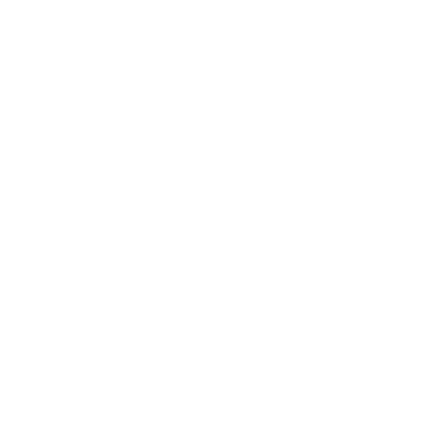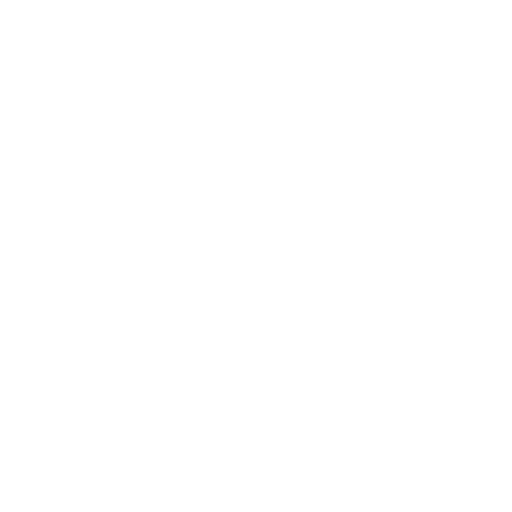# Free online number tools - numbermagics.com

## Simple, free and easy to use number utilities

•Generate Arithmetic Sequence
Arithmetic Sequence
•Generate Geometric Sequence
Geometric Sequence
•Draw Pascal Triangle
Pascal Triangle
•Convert Numbers to Picture
Numbers to Picture
•Generate Random Numbers
Generate Random Numbers
•Generate random prime numbers
Random Prime Numbers
•Generate Random Matrices
Random Matrices
•Generate Random Vectors
Generate Random Vectors
•Generate pi digits
Generate pi digits
•Generate Prime Numbers
Generate Prime Numbers
••Tool for Calculate digit product
Calculate digit product
•Tool for Calculate digit sum
Calculate digit sum
•Tool for Calculate e digits
Calculate e digits
•Tool for Calculate fibonacci numbers
Calculate fibonacci numbers
•Tool for Calculate number divisors
Calculate number divisors
•Tool for Calculate number product
Calculate number product
•Tool for Calculate number sum
Calculate number sum
•Tool for Calculate phi digits
Calculate phi digits
•Tool for Calculate pi digits
Calculate pi digits
•Tool for Calculate prime factors
Calculate prime factors
•Tool for Calculate prime numbers
Calculate prime numbers
•Tool for Change number base
Change number base
•Tool for Check number palindrome
Check number palindrome
•Tool for Convert number to negadecimal
•Tool for Convert number to power of ten
Convert number to power of ten
•Tool for Convert number to power of two
Convert number to power of two
•Tool for Convert numbers to words
Convert numbers to words
•Tool for Convert words to numbers
Convert words to numbers
•Tool for Create number palindrome
Create number palindrome
•Tool for Create unicode fractions
Create unicode fractions
•Tool for Filter numbers
Filter numbers
•Tool for Find greatest common divisor
Find greatest common divisor
•Tool for Find maximum number
Find maximum number
•Tool for Find minimum number
Find minimum number
•Tool for Generate even numbers
Generate even numbers
•Tool for Generate fibonacci like series
Generate fibonacci like series
•Tool for Generate integers
Generate integers
•Tool for Generate look and say numbers
Generate look and say numbers
•Tool for Generate odd numbers
Generate odd numbers
•Tool for Generate powers of ten
Generate powers of ten
•Tool for Generate powers of two
Generate powers of two
•Tool for Generate sequence of cubes
Generate sequence of cubes
•Tool for Generate sequence of squares
Generate sequence of squares
•Tool for Generate unit matrix
Generate unit matrix
•Tool for Linear recurrence calculator
Linear recurrence calculator
•Tool for Matrix determinant calculator
Matrix determinant calculator
•Tool for Number primality test
Number primality test
•Tool for Pick a number
Pick a number
•Tool for Round a number
Round a number
•Tool for Shuffle digits
Shuffle digits
•Tool for Sort digits
Sort digits
•Tool for Sort numbers
Sort numbers